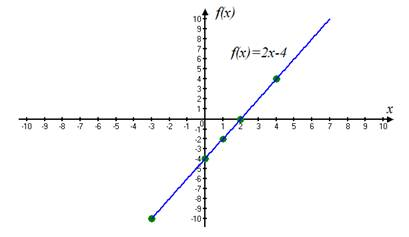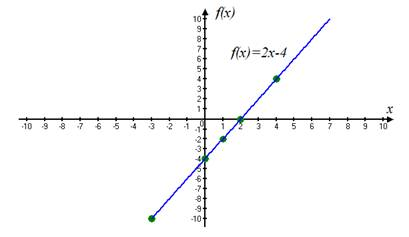# Sketch the graph of the function using table of values f ( x ) = 2 x − 4 .### Precalculus: Mathematics for Calcu...

6th Edition
Stewart + 5 others
Publisher: Cengage Learning
ISBN: 9780840068071### Precalculus: Mathematics for Calcu...

6th Edition
Stewart + 5 others
Publisher: Cengage Learning
ISBN: 9780840068071

#### Solutions

Chapter 2.2, Problem 7E
To determine

## Sketch the graph of the function using table of values f(x)=2x−4 .

Expert Solution

First make a table of values. Then plot the points given by the table and join them by a smooth curve to obtain the graph.### Explanation of Solution

Given information:consider the function f(x)=2x4 .

Calculation:

Let us take any values of x and then make a table of values.

xf(x)=2x432(3)4=1022(2)4=002(0)4=412(1)4=242(4)4=4

Then we plot the points given by the table and join them by a smooth curve to obtain the graph f(x)=2x4 as shown below:### Have a homework question?

Subscribe to bartleby learn! Ask subject matter experts 30 homework questions each month. Plus, you’ll have access to millions of step-by-step textbook answers!